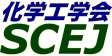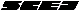## \$B9V1i%W%m%0%i%`!J2q>l!&F|DxJL!K(B

### L\$B2q>l(B \$BBh(B1\$BF|(B

\$B:G=*99?7F|;~!'(B2012-08-20 15:02:17
\$B9V1i(B
\$B;~9o(B
\$B9V1i(B
\$BHV9f(B
\$B9V1iBjL\!?H/I=\$B%-!<%o!<%I(B\$BJ,N`(B
\$BHV9f(B
\$B\$BHV9f(B
\$B%7%s%]%8%&%`(B \$B!c
(8:40\$B!A(B9:40)\$B!!(B(\$B:BD9(B \$B?\F#(B \$B2mIW(B)
8:40\$B!A(B 9:00L100PEFC\$BC1%;%k(Bin situ\$B29EYLLJ,I[B,Dj%G!<%?\$N:YJ,2=\$K\$h\$kEAG.FC@-I>2A\$HJ*
(\$B;0=EBg1!(B) \$B!{(B(\$B3X(B)\$B6aF#(B \$B90=S(B \$B!&(B (\$B@5(B)\$B@>B<(B \$B82(B \$B!&(B \$B;3Fb(B \$BBY2p(B \$B!&(B \$BHSHx(B \$BOB42(B \$B!&(B \$BW"ED(B \$B??;K(B
PEFC
In-plane temperature distribution
Impedance measurement
S-139
9:00\$B!A(B 9:20L101\$BM-5!(B-\$BL55!3&LL9bB.%W%m%H%sEAF38=>]\$rMxMQ\$7\$?8GBN9bJ,;R7AG3NAEECS\$N3+H/(B
(\$BEl9)Bg;q8;8&(B) \$B!{(B(\$B3X(B)\$B>.@n(B \$B7ILi(B \$B!&(B (\$B@5(B)\$BED4,(B \$B9'7I(B \$B!&(B (\$B@5(B)\$BBg66(B \$B=(Gl(B \$B!&(B (\$BElBg9)(B) \$B5m;3(B \$B9@(B \$B!&(B (\$B@5(B)\$B;32<(B \$B980l(B \$B!&(B (\$BEl9)Bg;q8;8&(B) (\$B@5(B)\$B;38}(B \$BLT1{(B
Fuel Cell
Proton conduction
an initio
S-153
9:20\$B!A(B 9:40L102\$B>.7?5!4oMQ(BPEFC\$B%9%?%C%/\$N7ZNL2=5Z\$SDc%3%9%H2=\$N8!F\$(B
(\$B90A0BgM}9)(B) \$B!{(B(\$B3X(B)\$BD%(B \$BD6(B \$B!&(B (\$B@D?98)9)Am8&(B) (\$B6&(B)\$B3k@>(B \$BM5(B \$B!&(B (\$B%S%C%H%F%C%/(B) \$BLZB<(B \$B1QM:(B \$B!&(B (\$B90A0Bg?7%(%M8&(B) (\$B@5(B)\$B41(B \$B9q@6(B \$B!&(B (\$B90A0BgM}9)(B) (\$B6&(B)\$B0KF#(B \$B> \$B!&(B (\$BElBg@88&(B) (\$B@5(B)\$BDi(B \$BFX;J(B \$B!&(B (\$B90A0Bg?7%(%M8&(B) (\$B@5(B)\$B0\$I[(B \$BN\$Ds(B

S-1110
(9:40\$B!A(B11:00)\$B!!(B(\$B:BD9(B \$B@>B<(B \$B82(B)
9:40\$B!A(B 10:00L103\$B8GBN9bJ,;R7AG3NAEECS1?E>;~\$N2a;@2=?eAG@8@.\$NI>2A(B
(\$B@EBg9)(B) \$B!{(B(\$B3X(B)\$B2,K\(B \$BM4 \$B!&(B (\$B3X(B)\$B>.Ln(B \$B8-;VO:(B \$B!&(B (\$B@5(B)\$B?\F#(B \$B2mIW(B
hydrogen peroxide
PEMFC
S-1354
10:00\$B!A(B 10:20L104\$BJ#6K@-Kl\$rMQ\$\$\$kG3NAEECS\$N1?E>FC@-(B
(\$B@EBg9)(B) \$B!{(B(\$B3X(B)\$B1J0f(B \$B=(OB(B \$B!&(B (\$B3X(B)\$B2<@n(B \$BN<2p(B \$B!&(B (\$B@EBg(B) (\$B@5(B)\$B?\F#(B \$B2mIW(B
Bipolar membrane
PEMFC
Plasma-Polymerization
S-1416
10:20\$B!A(B 10:40L105The Catalytic Properties of Nanoparticled Tantalum-based Catalysts by Electrodeposition under a Nonaqueous Plating Bath for Non-platinum Cathodes of PEFCs
(U. Tokyo) \$B!{(B(\$B3X(B)Seo J. \$B!&(B (KAUST) (\$B@5(B)Cha D. \$B!&(B (\$B@5(B)Takanabe K. \$B!&(B (U. Tokyo) (\$B@5(B)Kubota J. \$B!&(B (\$B@5(B)Domen K.
PEFC
Electrodeposition
Ta-based catalysts
S-1491
10:40\$B!A(B 11:00L106\$BJ.L84%AgK!\$KCf6u%]!<%i%9%+!<%\%sN3;R\$N9g@.\$HG3NAEECS?(G^\$X\$N1~MQ(B
(\$B9-Bg1!9)(B) \$B!{(B(\$B@5(B)\$B2.(B \$B?r(B \$B!&(B Ratna Balgis \$B!&(B (\$B@5(B)\$B1|;3(B \$B4n5WIW(B \$B!&(B (\$B%N%j%?%1%+%s%Q%K!<%j%_%F%I3+H/5;=QK\It(B) G. M. Anilkumar \$B!&(B \$B:89g(B \$B@!?M(B
Proton exchange membrane fuel cell
Electrocatalyst
spray drying
S-1638
(11:00\$B!A(B12:00)\$B!!(B(\$B;J2q(B \$BED4,(B \$B9'7I(B)
11:00\$B!A(B 12:00L107[\$B>7BT9V1i(B] \$BF|;:<+F0u\$HE8K>(B
(\$BF|;:<+F0
Polymer electrolyte fuel cell
S-1459
(13:00\$B!A(B14:20)\$B!!(B(\$B:BD9(B \$BJ!D9(B \$BGn(B)
13:00\$B!A(B 13:20L113\$BC:2=?eAG7OEE2r
(\$BEl5~;TBg(B) \$B!{(B(\$B3X(B)\$B6b;R(B \$BBYN4(B \$B!&(B \$BD9C+@n(B \$BK-(B \$B!&(B \$BFbCS(B \$BAo;V(B \$B!&(B (\$B@5(B)\$BIp(B \$BE/IW(B
Polymer Electrolyte Fuel Cell (PEFC)
Silica
Hydrocarbon membrane
S-1661
13:20\$B!A(B 13:40L114\$B0[\$J\$k%7%j%+8;\$K\$h\$jD4@=\$7\$?%7%j%+HoJ\$(BCNT\$BC4;}(BPt\$B%+%=!<%I?(G^\$N3h@-\$HBQ5W@-(B
(\$B6eBg1!9)(B) \$B!{(B(\$B3X(B)\$B1'ET5\(B \$BM5(B \$B!&(B \$B5\K\(B \$BBsL@(B \$B!&(B (\$B@5(B)\$BC]Cf(B \$BAT(B \$B!&(B (\$B@5(B)\$B>>:,(B \$B1Q \$B!&(B (\$B@5(B)\$B4_ED(B \$B>;9@(B
Pt catalyst
fuel cell
silica
S-1667
13:40\$B!A(B 14:00L115PEFC\$BMQ%+!<%\%s%J%N%A%e!<%VC4;}(BPd\$B%+%=!<%I?(G^\$NA+0\6bB0E:2C\$K\$h\$k?(G^3h@-8~>e(B
(\$B6eBg1!9)(B) \$B!{(B(\$B3X(B)\$BDMK\(B \$BCR@2(B \$B!&(B (\$B@5(B)\$BC]Cf(B \$BAT(B \$B!&(B (\$B@5(B)\$B>>:,(B \$B1Q \$B!&(B (\$B@5(B)\$B4_ED(B \$B>;9@(B
PEFC
Pd alloy catalyst
oxygen reduction reaction
S-1685
14:00\$B!A(B 14:20L116\$B%W%m%H%sEAF3@-%j%s;@%,%i%9\$N9g@.\$HEE5\$EAF3FC@-(B
(\$BElBg1!?7NN0h(B) \$B!{(B(\$B3X(B)\$B@nB<(B \$BN \$B!&(B (\$B@5(B)\$BBgM'(B \$B=g0lO:(B \$B!&(B (\$B@5(B)\$BBgEg(B \$B5A?M(B
fuel cell
proton conducting glass material
sol-gel method
S-1765
(14:20\$B!A(B15:40)\$B!!(B(\$B:BD9(B \$BC]Cf(B \$BAT(B)
14:20\$B!A(B 14:40L117Nafion\$B%-%c%C%T%s%0BN\$rMQ\$\$\$?EE6K?(G^AX\$N?(G^MxMQN(8~>e\$K8~\$1\$?8!F\$(B
(\$BEl9)Bg;q8;8&(B) \$B!{(B(\$B3X(B)\$BCfEg(B \$BC#:H(B \$B!&(B (\$B@5(B)\$BED4,(B \$B9'7I(B \$B!&(B (\$B@5(B)\$BBg66(B \$B=(Gl(B \$B!&(B (\$B@5(B)\$B;38}(B \$BLT1{(B
PEFC
Catalyst layer
Nafion Capping
S-1820
14:40\$B!A(B 15:00L118\$B%"%s%b%K%"=hM}\$7\$?%7%k%/3h@-C:\$r%+%=!<%I\$KMQ\$\$\$?(BPEFC\$B\$NH/EEFC@-(B
(\$B?.=#BgA!0](B) \$B!{(B(\$B@5(B)\$BJ!D9(B \$BGn(B \$B!&(B \$BBg;3(B \$B63J?(B \$B!&(B (\$B@5(B)\$B9b66(B \$B?-1Q(B \$B!&(B (\$B@5(B)\$B9bDM(B \$BF)(B \$B!&(B (\$BBgF|K\0u:~(B) (\$B@5(B)\$B4_K\(B \$BHfO\$;V(B
PEFC
non-precious metal catalysts
carbon
S-1956
15:00\$B!A(B 15:20L119\$B9g6bI=LL\$KD>@\9g@.\$7\$?%+!<%\%s%J%N%A%e!<%V\$N%0%k%3!<%9G3NAEECSEE6K\$X\$N1~MQ(B
(\$B5~Bg9)(B) \$B!{(B(\$B3X(B)\$B;y6L(B \$B9dIp(B \$B!&(B (\$B@5(B)\$B:4Ln(B \$B5*>4(B \$B!&(B (\$B@5(B)\$BEDLg(B \$BH%(B
fuel cell
carbon nanotube
chemical vapor deposition
S-17
15:20\$B!A(B 15:40L120A Facile Thermal Method for Producing Novel 3-D Graphite Oxide-Nanoribbon Supported Metal Electrocatalysts for DMFC
(\$BEl9)Bg;q8;8&(B) \$B!{(B(\$B3X(B)\$B]j(B \$B3\$NS(B \$B!&(B (\$B@5(B)Kakade B. A. \$B!&(B (\$B@5(B)\$BED4,(B \$B9'7I(B \$B!&(B (\$B@5(B)\$BBg66(B \$B=(Gl(B \$B!&(B (\$B@5(B)\$B;38}(B \$BLT1{(B
DMFC
methanol oxidation reaction
graphite oxide, nanoribbon
S-1298
(15:40\$B!A(B17:00)\$B!!(B(\$B:BD9(B \$B;38}(B \$BLT1{(B)
15:40\$B!A(B 16:00L121\$B9bG;EY%a%?%N!<%k\$r;HMQ\$9\$kD>@\%a%?%N!<%k7?G3NAEECS\$NH/EEFC@-(B
(\$B72Bg1!9)(B) \$B!{(B(\$B3X(B)\$B]/0f(B \$B=!(B \$B!&(B (\$B@5(B)\$BDT8}(B \$BBsLi(B \$B!&(B (\$B@5(B)\$BCf@n(B \$B?B9%(B
direct methanol fuel cell (DMFC)
boiling point
air humidity
S-1302
16:00\$B!A(B 16:20L122\$B%O%\$%Q!<%V%i%s%A%]%j%^!<\$rMQ\$\$\$?D>@\%.;@7?G3NAEECS\$NEE6K:n@=J}K!\$N8!F\$(B
(\$B72Bg1!9)(B) \$B!{(B(\$B@5(B)\$BDT8}(B \$BBsLi(B \$B!&(B (\$B3X(B)\$B4d>e(B \$BM@6k(B \$B!&(B (\$B3X(B)\$B>.V:(B \$BL((B \$B!&(B (\$B@5(B)\$BCf@n(B \$B?B9%(B \$B!&(B (\$BF|;:2=3X(B) \$B>.Eg(B \$B7=2p(B
DFAFC
Hyper branched-polymer
Stablizer
S-1458
16:20\$B!A(B 16:40L123\$BD>@\%.;@7?G3NAEECS\$NFC0[\$J%+%=!<%I2aEE05\$NA}2C(B
(\$B72Bg1!9)(B) \$B!{(B(\$B3X(B)\$B4d>e(B \$BM@6k(B \$B!&(B (\$B@5(B)\$BDT8}(B \$BBsLi(B \$B!&(B (\$B@5(B)\$BCf@n(B \$B?B9%(B
DFAFC
Cathode overvoltage
Low concentration
S-1561
16:40\$B!A(B 17:00L124TiO2\$B4^M-(BCNF\$BC4;}(BPtRu\$B?(G^\$N%"%k%3!<%k;@2=FC@-(B
(\$B72Bg1!9)(B) \$B!{(B(\$B3X(B)\$B0KF#(B \$BM:Bg(B \$B!&(B (\$B@5(B)Abdelkareem Mohammad Ali \$B!&(B (\$B@5(B)\$BDT8}(B \$BBsLi(B \$B!&(B (\$B@5(B)\$BCf@n(B \$B?B9%(B
DAFC
catalyst
TiO2
S-1679
(17:00\$B!A(B18:20)\$B!!(B(\$B:BD9(B \$BCf@n(B \$B?B9%(B)
17:00\$B!A(B 17:20L125\$BM}A[E*(BOH-\$BEAF3%A%c%M%k\$NC5:w\$K8~\$1\$?J,;R%G%6%\$%s7?5!G=@-:`NA\$N3+H/(B
(\$BEl9)Bg;q8;8&(B) \$B!{(B(\$B3X(B)\$BD9C+@n(B \$B00(B \$B!&(B \$B>1;R(B \$BNI98(B \$B!&(B \$BJ!Eg(B \$B9'E5(B \$B!&(B (\$B@5(B)\$BBg66(B \$B=(Gl(B \$B!&(B (\$B@5(B)\$BED4,(B \$B9'7I(B \$B!&(B (\$B@5(B)\$B;38}(B \$BLT1{(B
SAFC
micro phase separation
anion exchange membrane
S-1823
17:20\$B!A(B 17:40L126\$BA48GBN7?%"%k%+%jG3NAEECSMQEE2r
(\$BEl9)Bg;q8;8&(B) \$B!{(B(\$B3X(B)\$BCz(B \$B9aH~(B \$B!&(B (\$B@5(B)\$BBg66(B \$B=(Gl(B \$B!&(B (\$B@5(B)\$BED4,(B \$B9'7I(B \$B!&(B (\$B@5(B)\$B;38}(B \$BLT1{(B
fuel cell
Layered double hydroxide
anion conductor
S-1836
17:40\$B!A(B 18:00L127\$B:Y9&%U%#%j%s%07?%"%K%*%s8r49Kl\$NFC@-\$HH/EE;~?e0\F0\$N2r@O(B
(\$BEl9)Bg;q8;8&(B) \$B!{(B(\$B3X(B)\$BD%(B \$B^>(B \$B!&(B (\$B@5(B)\$BBg66(B \$B=(Gl(B \$B!&(B (\$B@5(B)\$BED4,(B \$B9'7I(B \$B!&(B (\$B@5(B)\$B;38}(B \$BLT1{(B
Anion exchange membrane
Water movement
Pore filling membrane
S-1847
18:00\$B!A(B 18:20L128\$BCf29:nF0%W%m%H%sEAF37?G3NAEECS\$K\$*\$1\$k3F
(\$BElBg1!?7NN0h(B) \$B!{(B(\$B3X(B)\$BEhED(B \$B8^I4N\$(B \$B!&(B (\$B@5(B)\$BBgEg(B \$B5A?M(B \$B!&(B (\$B@5(B)\$BBgM'(B \$B=g0lO:(B
intermediate temperature fuel cell
proton conductor
anode reaction
S-1547

\$B9V1i%W%m%0%i%`(B
\$B2=3X9)3X2q(B \$BBh(B44\$B2s=)5(Bg2q(B(C) 2012 \$B8x1W
Most recent update: 2012-08-20 15:02:17
For more information contact \$B2=3X9)3X2qElKL;YIt(B \$BBh(B44\$B2s=)5(Bg2q(B \$BLd\$\$9g\$;78(B
E-mail: inquiry-44fwww3.scej.org
This page was generated byeasp 2.28; proghtml 2.28c (C)1999-2011 kawase